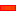﻿ Step 15.1: Piggyback Sliders

# Step 15.1: Piggyback Sliders

### Piggyback Sliders to model the Path of a Point on the Belt

We must model the motion of a point on the path of a Belt.

To do this, we design two motions for the Point: its X-axis and Y-axis motion components.

We give the each motion to a different Slider. We configure the two sliders as 'Piggyback Sliders'.

When we cycle, the Y-axis Slider moves in the Y-axis direction. The Y-axis Slider is being carried by the X-axis Slider. Thus, the Point also move in the X-axis direction.

If we design the motions correctly, the Point on the Y-axis Slider has the same motion as a Point on a Belt.

In this Tutorial Step, we add a set of Piggyback Sliders. We call the two Sliders a Slider Set.

We design the two motions for Slider-X and Slider-Y in Tutorial Step 15.2.

Note - From Release 11+, it is easier to use a sketch-loop and Motion-Path FB. See Also: Tutorial 11.

However, we should learn how we go about the model the piggyback sliders, as it is an important modelling method.

####About Piggyback Sliders

 What is a Piggyback? I am not sure if it is only an English term. When someone is off the ground and on the back of a standing person, and their hands are around the standing person's neck (to hold on, not to strangle!) and their legs are on each side of the standing person with their legs held up by the standing person's arms, then the person that is off the ground is being given a 'Piggyback'. The motion of the person on the back is a combination of their own motion and the motion of the person on the ground. Why Piggyback Sliders? We can design a motion for a Point to move along the X direction and a different motion to move along the Y direction, The total motion give the motion of a Point and Plane that moves (translates ) in the XY Plane. The total motion of the Slider that is supported = Motion Slider 1 + Motion Slider 2. Why not Piggyback Rockers? Yes we can have Piggy-Back Rockers also - this is exactly like a SCARA robot - see below. However, it is almost impossible to predict exactly what the motion of the Point at the end of the second Rocker will be if we give the motions, in angle [degrees], to the Rockers only. (Note: we can still design a motion for the XY Plane for a SCARA robot in MechDesigner. In fact we use Piggyback Sliders, as a simple trick.) New thoughts from the author since writing the above Piggyback Sliders Configuration. We add a Slider to the Base-Part that moves along the Y-axis of the Base-Part. (Slider-Y). Add Slider-X to Slider-Y in the -Y-axis direction of the Slider-Y (in the horizontal direction of the Base-Part). A Point in Slider-X with coordinates (x,y) has coordinates (X+x, Y+y) in the Base-Part.

#### How do we add Piggyback Sliders? Short Version.

We add the Piggyback Sliders as follows:

 • Add a Slider to move parallel to the X-axis of the Base-Part. We name it Slider-X
 • Edit Slider X to add vertical Line, usually along Y-axis of Slider X.
 • Add another Slider to the new Line in Slider-X to move along Y-axis of Slider-X. We name it Slider-Y.

A Point, called Point-XY, is a child to the Slider-Y.

The movement of the Piggyback Sliders and the Point XY, is as follows:

 • If only Slider-X moves, then Point-XY moves along the X-axis of the Mechanism-Plane, (or the Part Plane of the Part to which we join Slider-X).
 • If only Slider-Y moves, then Point-XY moves along the Y-axis of the Mechanism-Plane, (or the Part Plane of the Part to which we join Slider-X).
 • If Slider-X and Slider-Y move, then Point-XY moves with the combined movement in the Mechanism-Plane (or the Part Plane of the Part to which we join Slider-X).

####Add Piggyback Sliders - Long Version

The X-Slider will move along the X-axis of the Mechanism-Plane . It is the horizontal motion of the point on the belt.STEP 1: Edit the Base-Part; Add a Horizontal Line; Close the Part-Editor

To add the Line, drag from Left-to-Right. The positive direction of the Line will agree with the positive X-axis of the Base-Part.

Drag from Left-to-Right to add the Part. Add the Part adjacent to the Line. The positive direction of the Part agrees with the positive X-axis of the Base-Part.

 STEP 3: Add a Slide-Joint between the Part and the Line added to the Base-Part
 STEP 4: Add a Motion-Dimension FBto identify the Part as X-SliderThe positive direction of the X-Slider and Motion-Dimension is to the right. Edit the Base-Value of the Motion-Dimension

Edit the X-Slider, Add a Vertical LineSTEP 1: Edit the X-Slider Part

Edit the Length of the CAD-Line to 100mm - my habit.

 STEP 2: Add a Line[it is collinear with the Y-axis]

Drag from Bottom-to-Top to add the Line. The positive direction of the Line agrees with the positive Y-axis of the X-Slider Part.

 STEP 3: Add a Vertical Constraint to the Line
 STEP 4: Dimension and add Constraints to give the length and be coincident with the Y-axis

The length of the Line is 50. The Origin of the Line is Coincident, not merged, with the Origin of the X-Slider.

 STEP 5: Close the Part-Editor

The Y-Slider will move in the Y-axis of the XY-Plane. It is the vertical motion component of the point on the belt.STEP 3: Add a Motion-Dimension FBto identify the Part as Y-Slider